# Invariant average

invariant mean, on a group or semi-group, more precisely, an invariant mean or average on a spaceof functions onA continuous linear functionalon a closed subspaceof the space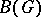of all bounded complex-valued functions on, endowed with the supremum norm, that contains the constant functions and is invariant under the operation of complex conjugation, and whereandsatisfy the following conditions: 1)is invariant under left translation, that is, if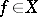then so is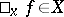, wherefor all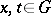,; 2)is a mean on, that is,for allandfor all real-valued; and 3)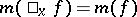for alland all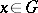. In this case the invariant meanis called a left-invariant mean; right-invariant and two-sided invariant means onare defined similarly.
If there exists on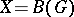a two-sided invariant mean, then the groupis called amenable. Amenability of a groupis related to the existence of invariant measures (cf. Invariant measure) with respect to certain transformation groups related to(see ). Ifis a locally compact topological group, then there exists on the spaces of almost-periodic functions and weakly almost-periodic functions ona non-trivial left-invariant mean. On the other hand, the following conditions are equivalent: 1) there exists a left-invariant mean on the space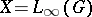; 2) there exists a left-invariant mean on the spaceof bounded continuous complex-valued functions on; 3) there exists a left-invariant mean on the space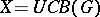of uniformly-continuous bounded complex-valued functions; 4) there exists a two-sided invariant mean on one of the spaces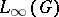,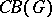,; 5) the groupdoes not have a complementary series (of representations); 6) the support of the regular representation ofcoincides with the dual space of; 7) the unit function oncan be uniformly approximated on any compactum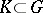by finite linear combinations of matrix entries of the regular representation of; 8) ifis a left Haar measure onandis a bounded complex-valued regular Borel measure onsuch that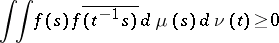for all continuous functionswith compact support in, then; 9) there exists for some,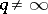, any, and any compactuma non-negative function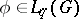,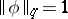, such that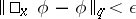for all; 10) the preceding condition holds for all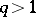,; 11) there exists for anyand any compactuma Borel setsuch that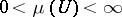and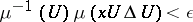for all; and 12) any continuous action ofon a compact convex subset of a locally convex space by continuous affine transformations has a fixed point. A locally compact group satisfying any of the equivalent conditions 1)–12) is called amenable. Continuous images of amenable groups, closed subgroups of amenable groups, extensions of amenable groups by amenable groups, and inductive limits of amenable groups are amenable. A uniformly-bounded representation of an amenable group in a Hilbert space is equivalent to a unitary representation in the same space. Some of the results listed above can be extended to the case of general topological groups admitting an invariant mean on the space of bounded continuous complex-valued functions. The theory of invariant means and amenable groups has important applications in the theory of dynamical systems, ergodic theory, the theory of von Neumann algebras, and abstract harmonic analysis (cf. also Harmonic analysis, abstract).